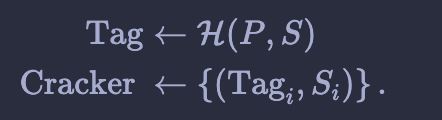简介

Memory hard function简称为MHF，在密码学中，内存困难函数(MHF)是一个需要花费大量内存来完成的函数。MHF主要被用在工作量证明中。因为需要花费大量的内存，所以MHF也会被用在密码Hash中，可以防止恶意破解。

MHF的种类

dMHFs的例子是scrypt和Argon2d。iMHFs的例子是Argon2i和catena。

MHF的密码学意义$\mathcal{E}_{\max }=\max _{\alpha} \frac{1}{\alpha D(\alpha)}$

memory-hard在MHF中的应用

$B[j]=G\left(B\left[\phi_{1}(j)\right], B\left[\phi_{2}(j)\right], \cdots, B\left[\phi_{k}(j)\right]\right)$

$\mathcal{E}(\alpha)=\frac{1}{\alpha+C(\alpha) \beta}$

$\mathcal{E}(\alpha)=\frac{1}{(\alpha+C(\alpha) \beta) D(\alpha)}$

posted @ 2021-05-26 08:05  flydean  阅读(273)  评论(0编辑  收藏  举报